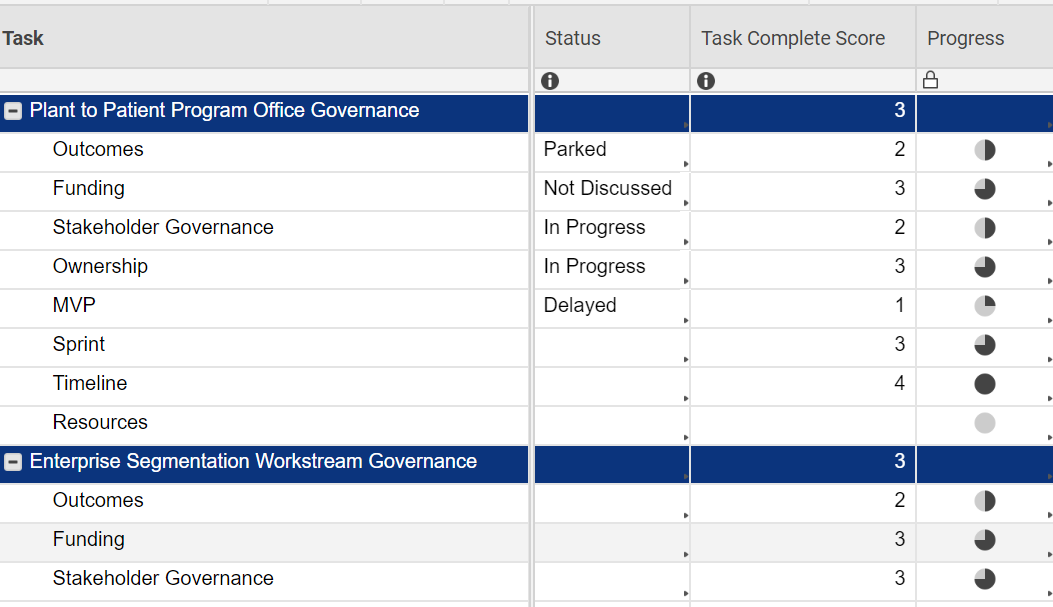or Explore Discussions

# Harvey Progress Ball at Parent Level

07/31/19 Edited 12/09/19

I have set up a column ("Task Complete Score") for numbering (0-4) to show progress which I am using to automate a column ("Progress") showing Harvey balls.    I’d like to add a Harvey Ball for the parent row using an easy average.  At the parent level, I have added this formula AVG(CHILDREN()) to get the average "Task Complete Score".  My formula to automate the Harvey Ball is not working on the Parent row even though a 0, 1, 2, 3 or 4 is returned by the formula.

Harvey ball formula:

IF([Task Complete Score]@row = 0, "Empty", IF([Task Complete Score]@row = 1, "Quarter", IF([Task Complete Score]@row = 2, "Half", IF([Task Complete Score]@row = 3, "Three Quarter", IF([Task Complete Score]@row = 4, "Full")))))

Any ideas?Popular Tags:

• That's because even though 3 is displayed, the ACTUAL result is 2.######.

Try using a ROUNDUP (or down or whatever suits your needs) to round it to the nearest whole number.

.

You could even use an INT function to just pull the whole number value.

0 - 0.99999 would equal 0, 1.0 - 1.99999 would equal 1, so on and so forth.